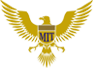Mathematics

MIT students make connections to the professional world in their math courses through challenges requiring them to solve problems in class and in the broader arena. The curriculum inculcates the following five process standards essential for developing deep understanding of mathematics: problem solving, reasoning and proof, communication, connections, and representation.

The math courses follow a sequential pathway, laying the foundation for further mathematical study. Each course has a strong emphasis on applied problems and integrates engineering curriculum throughout the content.

Course Offerings

• Pre-AP Algebra 1, 2
• Pre-AP Geometry
• Pre-Calculus/Trig
• AP Calculus AB/BC
• AP Statistics
• Multivariable Calculus/Differential Equations